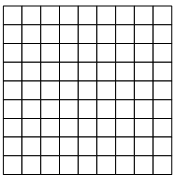In part 1 we looked at the method used for creating terrain. Midpoint Displacement is a fractal method which results in self-similarity implicitely making it scalable. Moreover the method can be scaled depending on the extent of the scope (e.g. 10 m vs 1 km vs 10 km), and number of iterations into smaller sub-divisions it is continued for (e.g. from 1 km to about 1m after 10 iterations). But imagine a large map of 1000 km, where we want to evaluate terrain upto a detail of 1 m, would take a lot of computing power, and significant storage. And this is only considering a single plane in 2 dimensions. So we can introduce partitioning. This is used in Minecraft, which uses three dimension segments of 16 x 16 (x 256) m.
In this case we use a two dimensional plane, of about 1000 km square, made up of 1000 x 1000 segments each representing 1 km square. Looking back to part 1, where we create an array of random seeds. Instead of a simple array, we create a 2 dimensional array of segements including a seed. To make this aspect scalable as well, seeds are generated in a similar order using a simple iterative midpoint method. This pattern is shown below, starting with the segments marked 1, then 2, 3, until each segment has a seed allocated.

step = size while step > 1
{
for y = 0:size:step
{
for x = 0:size:step
{
seed[y,x] = new seed
}
}
size / 2
}

Segmentation patternSegments are only evaluated on-demand. Each segement is initialised using its seed, generating a terrain map. In addition other characteristics are evaluated as we will see in part 3.